# How to Determine Tire/Wheel Diameter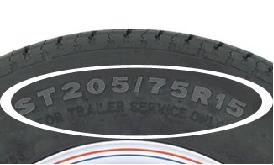If you want to know if a particular spare tire cover will fit, or are trying to find out how much clearance you would have when switching to another tire/wheel combo, there is an easy way to find out. Simply read the numbers off the sidewall of your current vehicle or trailer tire.

## Common Tire Size Parts

The most common tire sizes will have three parts, just like the 205/75-R15 tire pictured above. This number will tell you three things about the tire and wheel combination that you are trying to fit.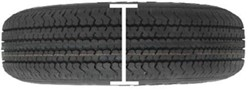### The First Number

The number 205 represents the section width of the tire, in millimeters. This is the measurement of the tire at its thickest point. If you want to convert centimeters to inches, divide the number of centimeters by 2.54. 1 Inch is about equal to 2.54 centimeters.

Example: 20.5 cm / 2.54 = 8.070 in

A 205/75-R15 tire would have a section width of 8.070 inches.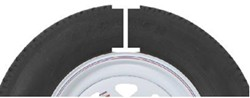### The Second Number

The number 75 refers to the sidewall aspect ratio. This will help you find out the height of the sidewall, from rim to tread. This is also called section height. The second number in a tire size indicates the ratio between tire width and height.

Example: 8.070 in x 0.75 = 6.053 in

A 205/75-R15 tire would have a section height that is 75 percent of 8.070 inches.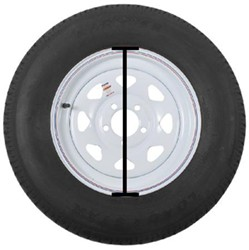### The Third Number

The last number, 15 in our example, represents the diameter, in inches, of the wheel on which the tire is mounted.

## Determining Total Diameter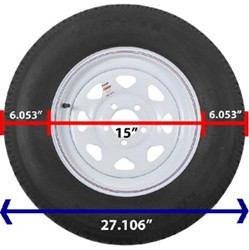Now that you know the section width, section height and rim size of the 205/75-R15 tire and wheel, you are able to determine the total diameter. The section height, or sidewall on the tire, is 6.053 inches. Multiply this by 2 to determine the amount of tire extending out on both sides of the wheel. Imagine drawing a straight line across the tire and wheel, directly across the center.

Example: 6.053 in x 2 = 12.106 in

Add the combined section heights of the tire (12.106 in) to the wheel size to get the combined diameter of the tire and wheel.

15 in + 12.106 in = 27.106 in

### Section Height

Section Height = Section Width x Aspect Ratio

### Tire and Wheel Diameters

Total Diameter = (Section Height x 2) + Wheel Diameter

## Exceptions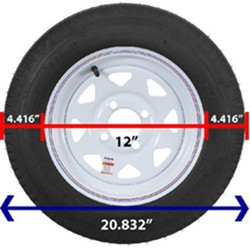Not every trailer tire will have a size like the example above. We also offer sizes such as 4.80-12 that do not automatically include all the information you need to determine the total tire and wheel diameter.

In the case of a size like 4.80-12, 4.80 is the section width of the tire in inches. The final number indicates the wheel diameter, similar to the more common tire size notation. If the first number of the size is 3 or 4 digits separated by a decimal point, you need to look at the last digit to determine the aspect ratio.

For numbers that end in 0, the aspect ratio is 92 percent. Numbers that do not end in 0 have an aspect ratio of 82 percent.

Example: 4.80 in x 0.92 = 4.416 in

A 4.80-12 tire has a section width of 4.8 inches, a section height of 4.416 inches and a wheel diameter of 12 inches. The combined diameter of the tire and wheel assembly is 20.832 inches.

Updated by: Raymond P.

Last updated: 7/3/18

## Contact & Help## What our customers are saying:

very easy to shop

Mike

Richmond, CA